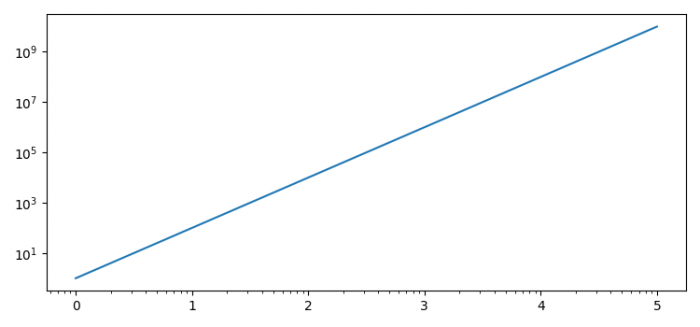# What is the correct way to replace matplotlib tick labels with computed values?

MatplotlibPythonData Visualization

We can use ax.loglog(x, y) and set_major_formatter() methods to replace tick labels with computed values.

## Steps

• Set the figure size and adjust the padding between and around the subplots.

• Create a figure and a set of subplots.

• Make a plot with log scaling on both the X and Y axis.

• Set the formatter of the major ticker.

• To display the figure, use show() method.

## Example

import numpy as np
from matplotlib import pyplot as plt, ticker

plt.rcParams["figure.figsize"] = [7.50, 3.50]
plt.rcParams["figure.autolayout"] = True

fig, ax = plt.subplots()

ax.loglog(np.logspace(0, 5), np.logspace(0, 5)**2)

ax.xaxis.set_major_formatter(ticker.LogFormatterExponent())

plt.show()

## Output